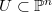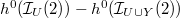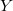﻿

### Postulation of double lines and associated objects in the range of quadrics

#### Abstract

Fix a closed subscheme$U\subset \mathbb {P}^n$. Here we study the integer$h^0(\mathcal {I}_U(2))-h^0(\mathcal {I}_{U\cup Y}(2))$ when$Y$ is a general double line, a general reducible conic, a general chain of lines or some unreduced structure associated to double structures on linear subspaces.

DOI Code: 10.1285/i15900932v31n2p79

Keywords: postulation ; Hilbert function ; unreduced schemes ; double lines ; quadric hypersurfaces

Full Text: PDF